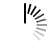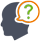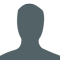Diwali Bonanza | Get 30-Days Free Fun-Filled Learning | Subscribe Now1800-833-6464 Buy Activation Why Tutorix Features Login

#DoubtTushar

In figure below, $Δ\ ACB\ ∼\ Δ\ APQ$. If $BC\ =\ 8\ cm$, $PQ\ =\ 4\ cm$, $BA\ =\ 6.5\ cm$ and $AP\ =\ 2.8\ cm$, find $CA$ and $AQ$.Solutions :Akhilesh

Given:

In the given figure $Î”\ ACB\ ∠¼\ Î”\ APQ$.

$BC\ =\ 8\ cm$, $PQ\ =\ 4\ cm$, $BA\ =\ 6.5\ cm$ and $AP\ =\ 2.8\ cm$.

To do:

We have to find the value of $CA$ and $AQ$.

Solution:

$Î”\ ACB\ ∠¼\ Î”\ APQ$  (given)

Therefore,

$\frac{BA}{AQ} = \frac{CA}{AP} = \frac{BC}{PQ}$   (Corresponding parts of similar triangles are proportional)

$\frac{6.5}{AQ} = \frac{8}{4}$

$AQ = \frac{6.5 \times 4}{8}$

$AQ = 3.25\ cm$

And,

$\frac{CA}{AP} = \frac{BC}{PQ}$

$\frac{CA}{2.8} = \frac{8}{4}$

$CA = 2.8 \times 2$

$CA = 5.6\ cm$

Hence, the values of $CA$ and $AQ$ are $5.6\ cm$ and $3.25\ cm$ respectively.

0

﻿